## Introduction

As building blocks for quantum computers, the implementation of quantum gates has received considerable research efforts over the recent years1. It has been reported experimentally that numbers of pulse-controlled microscopic systems, such as solid-state spins2 and trapped ions3, can be hosts for implementation of quantum gates. While enormous theoretical strategies for conventional quantum gate implementation have been proposed, there is a revived interest in using geometric phases to perform circuit-based quantum computation, termed as holonomic quantum computation (HQC)4, which is enabled by the adiabatic quantum theorem. The theorem asserts that at any instant a quantum system remains nearby in its instantaneous eigenstate of a slow-varying Hamiltonian, specifically for a cyclic adiabatic process, a geometric phase (the Berry’s phase), is acquired over the course of the cycle5. The geometric phase is exclusively determined by the trajectory of the system in its parameter space and robust against local fluctuation6,7. Consequently, a geometric strategy for implementation of quantum gates permits fault-tolerant and robust quantum information processing. Besides inherent resilience in non-Abelian geometric phases8, HQC has an appealing advantage9,10,11 in utilizing the state-of-art experimental setups due to its close relationship to the circuit model12,13,14. A recent experiment has implemented a universal set of geometric quantum logic gates with diamond nitrogen-vacancy centers15, and evidently it will greatly promote research endeavour along this line.

The heart of HQC is the experimental implementation of the geometric phase acquired in a cyclic adiabatic passage. Despite its advantages, the geometric protocol itself is challenged with a dilemma. On one hand, any HQC algorithm requires a long characteristic runtime in order to satisfy the adiabatic condition16. On the other hand, decoherence or leakage accumulated in this long runtime gives rise to errors in the HQC processing and may eventually destroy the quantumness of the system. To get rid of the dilemma, researchers have proposed several different protocols. Over a decade ago, Wu, Zanardi and Lidar17 initiated a scheme by embedding HQC into a decoherence-free subspace (DFS). This combined HQC-DFS scheme utilizes the virtues of both the fault-tolerance of HQC and the robustness of DFS against collective dephasing noise based on the symmetry structure of the interaction between the system and its environment. However, the residual individual noise remains and ruins the quantum adiabatic passages during the long runtime. Later on the HQC-DFS scheme was extended by considering the collective dephasing of two neighboring physical qubits18. Whereas it is more feasible experimentally, this scheme has a more stringent requirement for the runtime. Recently a non-adiabatic HQC-DFS scheme was suggested where the characteristic timescale is reduced by increasing the characteristic energy, at the cost of a harsh restriction for the runtime equal to the period of the system19. However, the fault tolerance from adiabaticity therefore becomes obscure.

In this paper, we propose a novel and composite strategy to tackle the long runtime issue in the HQC protocols via accelerating the adiabatic passage in DFS. We explain the mechanism and show specifically that the characteristic timescale of the adiabatic process can be vastly reduced by means of external field control20. Interestingly, it is found that the particular design or shape of a control function, such as regular, random, chaotic and even noisy pulse sequences, is not as decisive as it seems to be, but only the integral of the control function in the time domain plays the crucial role in speeding up the adiabatic passage, which greatly relaxes constraints on experimental implementation of these control functions. Remarkably, we further discover that our Hamiltonians in the adiabatic representation are periodical functionals of the integral of the control functions, resulting in a net zero-energy-cost control scheme – a new mechanism that accelerates adiabatic passages with the same effectiveness. These lead to a new type of fault-tolerance against control fluctuations.

## Results

### Decoherence-free subspace for qubit gates

Decoherence-free subspace is based on the symmetry structure of the system-environment interaction21,22,23,24,25. Here we briefly recall the method to realize a universal set of quantum gates acting on the DFS as firstly proposed in ref. 17. To implement a one-qubit quantum gate in DFS, we consider a four physical qubit system with the Hamiltonian, where,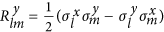are the XY interactions and Dzialoshinski-Moriya terms,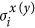is Pauli X(Y) matrix acting on the i-th physical qubit and m, l = 1, 2, 3, 4. This Hamiltonian commutes with the operator, where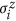is a Z Pauli matrix acting on i-th physical qubit. By setting, where φ(t) is specifically designed for HQC,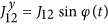,and all other, the Hamiltonian becomes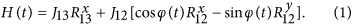The bases for DFS have been identified as eigenvectors of Z17, as spanned by {|0〉, |1〉, |2〉, |3〉}, where |0〉 = |0001〉 and |1〉 = |0010〉 constitute the two orthonormal states for a logical qubit and |2〉 = |1000〉 and |3〉 = |0100〉 serve as ancilla. This DFS scheme is robust against collective dephasing described by ZB, where B is an arbitrary Hermitian bath operator. It is straightforwardly proven that in the DFS, the Hamiltonian (1) can be rewritten as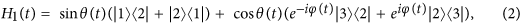where θ(t) = tan−1(J13/J12).

### Holonomic quantum computation in DFS

Consider a quantum system whose dynamics is governed by a time-dependent Hamiltonian H(t) with instantaneous eigenvectors |En(t)〉 and eigenvalues En(t). The wave function |ψ(t)〉 satisfies the Schrödinger equation and can be formally written as, where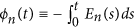is the dynamical phase. If the Hamiltonian varies adiabatically and there is a non-vanishing gap between the interested eigenvalues, the system will remain in the corresponding instantaneous eigenstate. Consequently, a Berry’s phase is given when the system passes along a closed loop in the Hamiltonian parameter space, which is path-independent. Without loss of generality, one can consider a case where the system is initially at the n-th ground state |En〉. It follows that in the adiabatic regime, where γn(t) is the Berry’s phase given by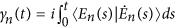. Here we emphasize that for dark states with eigenenergy En(t) = 0, its dynamical phase vanishes and the remaining overall phase is a geometric phase.

Equipped with Eq. (2), we are ready to construct our expedited-HQC-DFS scheme. To build up a one-qubit gate in DFS, we consider a cyclic Hamiltonian with period of T. We first consider a single qubit phase gate. The Hamiltonian H1(t) is formally given by Eq. (2) regarding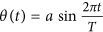,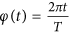, where a is a dimensionless undetermined coefficient. The two dark states in the DFS for Hamiltonian H1(t) read as |D0(t)〉 = |0〉 and, respectively.

In the adiabatic regime, under the unitary evolution, where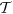is time-ordering operator, the dark states |D0〉 and |D1〉 become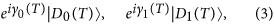respectively, where γj(T) is the Berry’s phase for |Dj〉, j = 0, 1. In this manner we achieve a one-qubit phase gate by. Note that |Dj(T)〉 = |Dj(0)〉. The gate can be expressed by a diagonal matrix as. The two Berry’s phases for dark states are γ0(T) = 0 andwhere J0(x) is a zero order Bessel function of the first kind, respectively.

This technique is also applicable in realization of a single σx qubit gate. To build this gate, we implement the Hamiltonian in the same DFS yet spanned by {|+〉, |−〉, |2〉, |3〉}, where. It is written as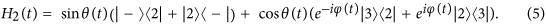In this case, the new dark states are |D0(t)〉 = |+〉 and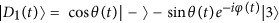, respectively. The transformations of dark states under time evolution are still described by Eq. (3), and the qubit gate reads,which becomes the σx-gate when γ1(T) = π. Now we turn to the two-qubit controlled-phase (C-Phase) gate in DFS. Since each logical qubit consists of four physical qubits, eight physical qubits are involved in implementing a two logical-qubit gate. Let us suppose that one can implement the HamiltonianThe four dark states of the Hamiltonian employed in implementing C-Phase gate are given by |D0(t)〉 = |0, 0〉, |D1(t)〉 = |0, 1〉, |D2(t)〉 = |1, 0〉,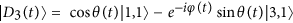, respectively.

Over a period T, the Hamiltonian (7) drives these states into |D0(0)〉 → |D0(T)〉, |D1(0)〉 → |D1(T)〉, |D2(0)〉 → |D2(T)〉 and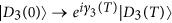, so that the two-qubit gate is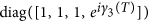, where γ3(T) = γ1(T) in Eq. (4). Tuning the free parameter a, one can get an arbitrary phase gate at will, for example, γ3(T) = π requires J0(2a) = 0 at the first root a = 1.2024.

### Control scheme

We now come to the case where the Hamiltonian H(t) is not in the adiabatic regime. Our scheme is to implement a control c(t) upon the strength of the Hamiltonian such that20,26We first show that as long as the control is sufficiently fast and strong (which will be specified later). the system evolution will behave in the same way as that in the adiabatic regime, specifically the wave function |ψ(t)〉 becomes proportional to an instantaneous eigenstate of H(t). It is interesting to note that this control scheme hardly depends on the details of c(t) but its integral in the time domain, and is a new type of fault-tolerance against control fluctuations. Consequently, the evolution of the corresponding dark states are shown to be a qualified workstation for HQC and this induced adiabaticity will be utilized to realized the expedited HQC in virtue of a fast modulation over Hamiltonian. We emphasize that the results given in Eq. (4) are invariant under the transformation (8), which is one of key points of our proposal.

To determine the effectiveness of our control scheme, we now introduce a quality factorwhere δγ1 is the difference between the ideal phase (4) and the phase acquired during a finite runtime T. Note, |δγ1| ≤ π because of the periodicity of the phase factor. Accordingly, due to our choice of quality factor (9) we have 0 ≤ f ≤ 1 where f = 1 if and only if the process is perfectly adiabatic and retain the Berry phase predicted by (4). Figure 1 shows f as a function of evolution time T (blue curve) in the absence of control (c(t) = 0), and as a function of average noise kick’s strength 〈c(t)〉 for T (red dashed curve) that is not in the adiabatic domain.

### Discussion: Expedited HQC with net zero energy cost

On closely looking into its pattern, we find that the Hamiltonian (2) in the adiabatic representation (see Methods) is eventually a functional of the exponent eiC(t), i. e.,. Because of the periodicity of eiC(t), our control scheme allows for an interesting case when 〈c′(t)〉 = 0, where c′(t) has alternating positive and negative values such that the net energy cost is zero. We first illustrate that the above-discussed positive control c(t) (with) can be exactly equivalent to zero-energy-cost control c′(t), when c(t) = πiδ(t − τi) with the integral C(t), and c′(t) = πi(−1)iδ (t − τi) with C′(t). It is easy to show that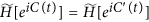due to the periodicity of eiC(t), and 〈c(t)〉 = 2πτiτi = τi+1 − τi) but 〈c′(t)〉 = 0 for each two consecutive pulses. The random intervals Δτi are much shorter than T in reality, and ideally the net energy cost of the c′(t) control sequence can be considered as zero when Δτi approaches zero. We can also analyze the equivalence for the rectangular pulses sequences. Based on the first order of Magnus27 expansion of U(δt) we can justify (see Methods) that if the single pulse strength, the off-diagonal terms in evolution U(δt) become zero whenIn Fig. 2 we show the numerical simulation of the quality factor f for fixed T = 10 with net zero-energy-cost control as a function of control pulse length Δt. We mark with triangles and squares when Eq. (10) is satisfied. The green solid curve in Fig. 2 shows the zero-energy-cost noise control which is more robust against the control “kick” length Δt, while noise positive control has prominent oscillatory dependence on Δt which requires a more accurate choice of Δt (and/or J) according to Eq. (10). In Fig. 3 we show in detail how the quality factor f depends on JΔt/2π for different J values. Solid (dotted) lines correspond to the noiseless (noise) control. From Fig. 3 we can see that one can achieve f > 1–10−4 which is necessary for practical quantum computation by increasing strength J for both noise and noiseless control (note, the increase of J doesn’t change the average 〈c(t)〉 = 0 in net-zero-energy control). This circumstance follows from (10) which is derived based on the assumption, meaning that the increase of J automatically implies the decrease of Δt when n = Const.

Both HQC and DFS have been experimentally demonstrated in different physical systems. For instance, HQC was realized in the nuclear magnetic resonance (NMR)28, trapped ions29 and superconducting qubit experiments30. DFS has been experimentally demonstrated in NMR31, trapped ions32 and photonic systems33. Generalization of the DFS idea to noiseless subsystems34 was experimentally tested in ref. 35. The technique to implement the strength as in our control scheme has been developed and used, for example, in superconducting qubit experiments in ref. 30 by applying microwave pulses with time-dependent envelope.

## Methods

To understand the mechanism of our expedited HQC scheme we expand the wave function in terms of eigenstates |En(t)〉 of the Hamiltonians. The matrix elements of the Hamiltonians in the adiabatic representation reads,20. For example, the Hamiltonian (2) is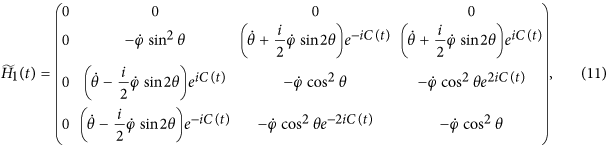where. It shows clearly that the Hamiltonian is a functional of the integral C(t) (or the average of c(t) in the time domain) i.e.,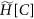, meaning that controlled dynamics does not depend on the details of c(t) but exclusively depends on the integral C(t). Such exclusive dependence also holds for our Hamiltonians (2), (5) and (7), and is a unique feature of our chosen Hamiltonians whose energy differences Enm = Em − En are time-independent constants. These Hamiltonians, as shown in its adiabatic representation, are incidentally equivalent to the Leakage Elimination Operators36. Hence, the control is fault tolerant in the sense that the fluctuation or noise of c(t) hardly contributes to C(t). More specially, by considering the propagator from t = 0 to t = δt, where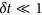and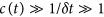, we can write the propagator as,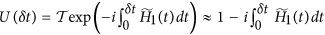. The existence of the fast oscillating factor eiC(t) renders all the off-diagonal elements of the propagator vanish and then leaves a Berry’s phase to the amplitudes of |D1〉 and two bright eigenstates. Noticeably this factor pushes the evolution of system into the adiabatic regime by decoupling all the four eigenstates. It clearly illustrates the advantage of our control scheme: one needs not to care about the exact control function because only the integral C(t) contributes to adiabaticity.

Expression (10) could be easily derived by the following consideration. We again consider short time evolution U(δt), and now we set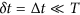, where Δt is control pulse length. Adiabaticity means that off-diagonal elements of the matrixare zero. Each of these off-diagonal elements could be written as, here we assume thatand f(t) is a smooth function: f(t) ≈ f(t + Δt), sign ± corresponds to the positive and negative control pulses. We can then conclude that off-diagonal elements of Ut) becomes close to zero when JΔt = 2πn (n = 1, 2, 3, …), i.e. we have Δt equals to integer numbers of periods 2π/J.

## Conclusion

To cope with the long runtime issue in implementing adiabatic passages, we have introduced an expedited-HQC-DFS control scheme to accelerate the conventional HQC. We show explicitly that the effectiveness of our control scheme exclusively depends on the integral of the external control functions in the time domain. Therefore the scheme is robust against stochastic errors in control. More importantly, we further find that the Hamiltonian in the adiabatic representation is a periodical functional of the integral of the control. The periodicity motivates us to design a net zero energy cost strategy for speedup which is also robust against control imperfections. These novel results are confirmed by numerical results. This observation greatly reduces the experimental constraints in generating precisely-shaped pulses and allows us to use even random pulse sequences. By combining the features of this scheme with a scalable DFS, our expedited HQC protocol brings together the advantages of all-geometrical HQC, decoherence-free subspace, zero-energy-cost control, and our fault tolerant scheme, a typical scalable, fast and fault-tolerant architecture. We therefore expect that this perfect theoretical protocol becomes an experimental practice.